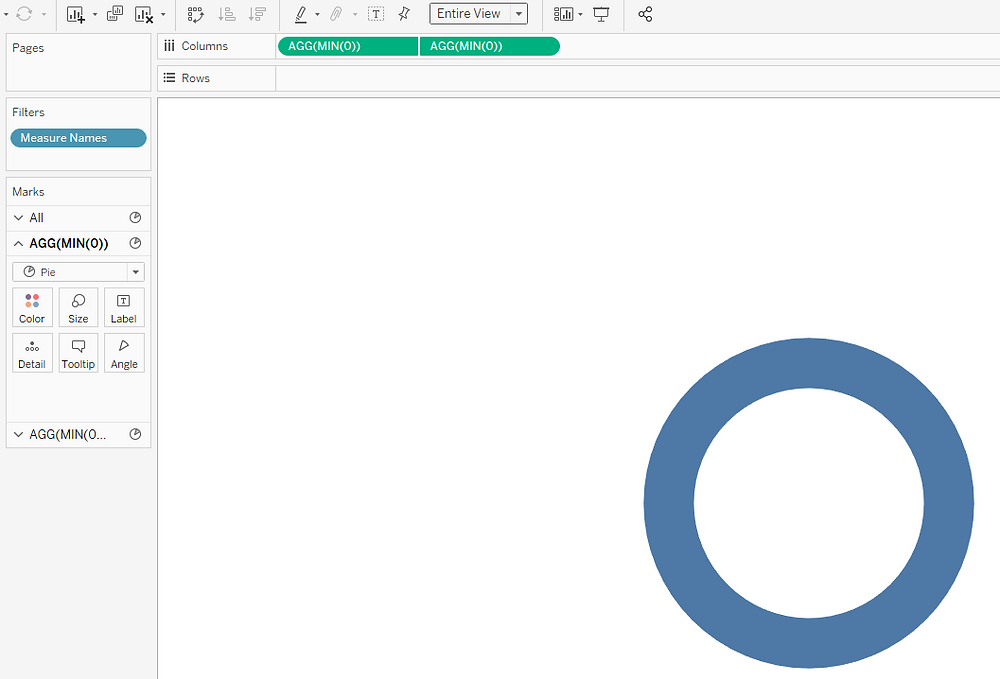Search

# How to create NPS Gauge Chart (Color-coded) in Tableau (No Custom Data)

Updated: Mar 6, 2020

Hello guys, welcome back again!

So the last time we created simple gauge charts in tableau without custom data, using only calculations.

Didn't read that one? Go through that here.

Now coming back to the topic, today we will be creating NPS (Net Promoter Score) gauge chart. A NPS gauge is simply an upgrade to the simple gauge chart as it visually represents the impact or outcome of the value in the form of color.

So lets start by creating a calculation - the value which we want to represent in the gauge. Any % value will do. Let's call it VALUE.

eg:

VALUE

SUM([SALES])/SUM([TARGET])

Set the default properties to percentage without any decimals.

The idea is to color the donut according to the value of the %.

If the value is less than 33%, it will be red.

If the value is more than 66%, it will be green.

If the value is between 33% and 66%, it will be yellow.

Step-1 : Creating Necessary Calculations

Now that we have it, lets start by creating the 10 calculations as follows:

NPS-1 Red

IF [VALUE]<=.33 THEN [VALUE] ELSE 0 END

NPS-2 Yellow

IF [VALUE]>.33 AND [VALUE]<=.5

THEN [VALUE]

ELSEIF [VALUE]>.5 AND [VALUE]<.=66

THEN .5

ELSE 0 END

NPS-3 Green

IF [VALUE]>.66 THEN 0.5 END

NPS-4 Grey

IF [VALUE]<=.5

THEN [VALUE]-.5

ELSEIF [VALUE]>.5 AND [VALUE]<.66

THEN 0

ELSE 0 END

NPS-5 Yellow

IF [VALUE]>.5 AND [VALUE]<=.66 THEN [VALUE]-.5 END

NPS-6 Green

IF [VALUE]>.66 THEN [VALUE]-.5 END

NPS-7 Grey

IF [VALUE]<=.5

THEN .5

ELSEIF [VALUE]>.5

THEN 1-[VALUE] END

NPS-8 White

MIN(.51)

Line1

IF [VALUE]>=.5 THEN .01 END

Line2

IF [VALUE]<.5 THEN .01 END

Dummy

'Dummy'

Step-2 : Creating the base

Now create a basic donut chart which will act as a base to your 'pizza'.

It should look something like this:Basic donut base - Check mark types

Step-3 : Selecting the measuresNow next step is to drag Measure Names into the filter pane and select the measures which you just created.

These measures will be then used to create the doughnut

...oopss, I mean the Gauge, HAHA!

Step-4 : Aligning the measures

Now we will put the Measure Names into color and Measure Values into Angle in both the marks cards. Make sure the measures are aligned in the following order:Also, ensure that the colors are as shown.

You will see something like this:Almost done...

Step-4 : Final Touch

Now put the Dummy field in the details of the inner pie. Click on the detail icon besides the Dummy field and select Color.

Now the Measure Names and Dummy will together create a temporary combined field.

Color them as :We only want to see the Needle/lines in the inner pie. Hence we will white-out the rest of the fields.

And now, after a few finishing touches,......

Hope you guys liked this one.

Did I miss something?

Do you believe it can be improved?

Let me know, suggestions are always welcome!

See you soon again!

Fin.